# Resources tagged with: Equally likely outcomes

Filter by: Content type:
Age range:
Challenge level:

There are 9 NRICH Mathematical resources connected to Equally likely outcomes, you may find related items under Probability.

Broad Topics > Probability > Equally likely outcomes### Connect Three

##### Age 11 to 16 Challenge Level:

In this game the winner is the first to complete a row of three. Are some squares easier to land on than others?### Cosy Corner

##### Age 11 to 14 Challenge Level:

Six balls are shaken. You win if at least one red ball ends in a corner. What is the probability of winning?### Two's Company

##### Age 11 to 14 Challenge Level:

Seven balls are shaken. You win if the two blue balls end up touching. What is the probability of winning?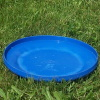### Flippin' Discs

##### Age 11 to 14 Challenge Level:

Discs are flipped in the air. You win if all the faces show the same colour. What is the probability of winning?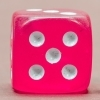### What Do You Know about Probability? (2)

##### Age 11 to 14 Challenge Level:

What are the likelihoods of different events when you roll a dice?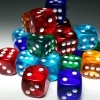### What Do You Know about Probability? (1)

##### Age 11 to 14 Challenge Level:

How can we use dice to explore probability?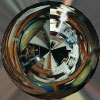### Misunderstanding Randomness

##### Age 11 to 14 Challenge Level:

Which of these ideas about randomness are actually correct?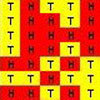### Can't Find a Coin?

##### Age 11 to 14 Challenge Level:

Can you generate a set of random results? Can you fool the random simulator?### Master Minding

##### Age 11 to 14 Challenge Level:

Your partner chooses two beads and places them side by side behind a screen. What is the minimum number of guesses you would need to be sure of guessing the two beads and their positions?